# MAFS.3.NF.1.1

Understand a fraction 1/b as the quantity formed by 1 part when a whole is partitioned into b equal parts; understand a fraction a/b as the quantity formed by a parts of size 1/b.
General Information
Subject Area: Mathematics
Domain-Subdomain: Number and Operations - Fractions
Cluster: Level 2: Basic Application of Skills & Concepts
Cluster: Develop understanding of fractions as numbers. (Major Cluster) -

Clusters should not be sorted from Major to Supporting and then taught in that order. To do so would strip the coherence of the mathematical ideas and miss the opportunity to enhance the major work of the grade with the supporting clusters.

Date of Last Rating: 02/14
Status: State Board Approved
Assessed: Yes
Test Item Specifications
Also assesses:
MAFS.3.G.1.2

• Assessment Limits :
Denominators are limited to 2, 3, 4, 6, and 8. Items are limited to combining or putting together unit fractions rather than formal addition or subtraction of fractions. Maintain concept of a whole as one entity that can be equally partitioned in various ways when working with unit fractions. Fractions a/b can be fractions greater than 1. Items may not use the term “simplify” or “lowest terms” in directives. Items may not use number lines. Shapes may include: quadrilateral, equilateral triangle, isosceles triangle, regular hexagon, regular octagon, and circle.
• Calculator :

No

• Context :
Allowable for 3.NF.1.1; no context for 3.G.1.2
Sample Test Items (5)
• Test Item #: Sample Item 1
• Question:

Each model shown has been shaded to represent a fraction. Which model shows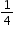shaded?

• Difficulty: N/A
• Type: MC: Multiple Choice

• Test Item #: Sample Item 2
• Question:

Each model shown has been shaded to represent a fraction. Which model shows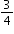shaded?

• Difficulty: N/A
• Type: MC: Multiple Choice

• Test Item #: Sample Item 3
• Question:

A figure is shown. Part of the figure is shaded.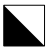Which fraction of the total area of the figure does the shaded part represent?

• Difficulty: N/A
• Type: EE: Equation Editor

• Test Item #: Sample Item 4
• Question:

A figure is shown. Part of the figure is shaded.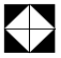Which fraction of the total area of the figure does the shaded part represent?

• Difficulty: N/A
• Type: EE: Equation Editor

• Test Item #: Sample Item 5
• Question:

Each shape shown represents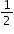of a whole.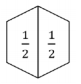How many shapes should be put together to make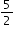?

• Difficulty: N/A
• Type: EE: Equation Editor

## Related Courses

This benchmark is part of these courses.
5012050: Grade Three Mathematics (Specifically in versions: 2014 - 2015, 2015 - 2022 (current), 2022 and beyond)
7712040: Access Mathematics Grade 3 (Specifically in versions: 2014 - 2015, 2015 - 2018, 2018 - 2022 (current), 2022 and beyond)
5020100: STEM Lab Grade 3 (Specifically in versions: 2016 - 2022 (current), 2022 and beyond)
5012055: Grade 3 Accelerated Mathematics (Specifically in versions: 2019 - 2022 (current), 2022 and beyond)
5012015: Foundational Skills in Mathematics 3-5 (Specifically in versions: 2019 - 2022 (current), 2022 and beyond)

## Related Access Points

Alternate version of this benchmark for students with significant cognitive disabilities.
MAFS.3.NF.1.AP.1a: Identify the number of highlighted parts (numerator) of a given representation (rectangles and circles).
MAFS.3.NF.1.AP.1b: Identify the total number of parts (denominator) of a given representation (rectangles and circles).
MAFS.3.NF.1.AP.1c: Identify the fraction that matches the representation of partitioned rectangles and circles into halves, fourths, thirds, and eighths.

## Related Resources

Vetted resources educators can use to teach the concepts and skills in this benchmark.

## Assessments

Sample 3 - Third Grade Math State Interim Assessment:

This is a State Interim Assessment for third grade.

Type: Assessment

Sample 2 - Third Grade Math State Interim Assessment:

This is a State Interim Assessment for third grade.

Type: Assessment

Sample 1 - Third Grade Math State Interim Assessment:

This is a State Interim Assessment for third grade.

Type: Assessment

## Educational Games

Fraction Quiz:

Test your fraction skills by answering questions on this site. This quiz asks you to simplify fractions, convert fractions to decimals and percentages, and answer algebra questions involving fractions. You can even choose difficulty level, question types, and time limit.

Type: Educational Game

Fraction Beach:

In this interactive Flash game, students are challenged to identify a fraction from a picture of a group of objects or from a geometric diagram, or they are asked to create a diagram or picture given a common fraction. Motivation is provided by earning buckets of sand to built a sand castle.

Type: Educational Game

## Educational Software / Tool

Crossing the River Online Game (Identifying Fractions):

This interactive, online game is a fun way for students to practice identifying fractions. In this lesson students identify fractions to help a man hop his way across a river.

Type: Educational Software / Tool

## Formative Assessments

Which Shows One Third?:

Students are shown three circles and asked to select the one that correctly shows one third shaded and explain why the other two do not.

Type: Formative Assessment

What Does One Fifth Mean?:

Students are shown the fraction one fifth and asked to describe what it means.

Type: Formative Assessment

Three Quarters Of The Race:

Students are read a word problem about a student who has run three-fourths of a race and asked to describe what that means.

Type: Formative Assessment

Painting A Wall:

Students are read a word problem about a wall being painted and asked to describe what three-eighths of the wall means.

Type: Formative Assessment

## Image/Photograph

Clipart ETC Fractions:

Illustrations that can be used for teaching and demonstrating fractions. Fractional representations are modeled in wedges of circles ("pieces of pie") and parts of polygons. There are also clipart images of numerical fractions, both proper and improper, from halves to twelfths. Fraction charts and fraction strips found in this collection can be used as manipulatives and are ready to print for classroom use.

Type: Image/Photograph

## Lesson Plans

Happy Feet!:

In this chocolately delicious lesson, your students will enjoy learning how to compare fractions and use that mathematical knowledge to create an inventory for a new local shoe store, Happy Feet Footwear. They will also write to express their opinions and provide reasons to justify their opinions.

This lesson suggests using "The Hershey's Milk Chocolate Fraction Book" to help students manipulate and compare fractions and have a great time doing so! What student wouldn't like to use chocolate to help him/her learn?

Type: Lesson Plan

Mystery Strips - Fractional Parts of the Whole:

This problem-solving lesson has students working together in groups to discover that fractions are made up of equal parts of one-whole. In this lesson, students are exposed to equivalent fractions as well as challenged to work beyond unit fractions to discover the fractional part of one-whole that the "mystery strips" represent. This highly engaging lesson takes into account all levels of learners and will challenge even your most advanced students.

Type: Lesson Plan

Parts of a Whole:

In this lesson, students will use area models to partition a whole into equal parts and record the associated fractions that represent the part/s. Students will determine and explain whether or not a given fraction and an area representation match. Various shapes will be used for the area models.

Type: Lesson Plan

Fraction Action!:

This lesson will help students understand that fractions are parts of a whole. The lesson introduces fractional parts using familiar manipulatives.

Type: Lesson Plan

Represent and Write Fractions:

Students will demonstrate their understanding of representing and recording fractions using pictures, manipulatives and numbers through an interactive problem solving scenario. Students will create a "picture pie" as an introduction to fractions and also will demonstrate the connection to the numerical form of a fraction and the picture form in a mix-match-mingle game.

Type: Lesson Plan

Fraction Face Off:

In this lesson, students will become familiar with comparing fractions and play a "war" game with fractions.

Type: Lesson Plan

Discovering Fractions:

In this lesson students will make initial discoveries about fractions. Students will work together to explain and record the discoveries they make while using manipulatives to explore fractions.

Type: Lesson Plan

Fraction Name Art:

This lesson is designed to introduce and give students practice with the concept of fractions as part of a set. Students will use their classmates to create fraction statements, play a guessing game with color tiles, and finally write fractional statements about their own Name Art!

Type: Lesson Plan

The "Whole" Deal:

This is an introductory fraction lesson designed to reinforce the idea that fractions are equal parts of one whole. The students will use different pattern blocks as their whole to increase their understanding of fractions in a variety of different representations.

Type: Lesson Plan

"What's the part? What's the whole?":

This lesson provides a conceptual approach to multiplying a fraction times a whole number and a whole number times a fraction.  Students are to use an understanding of the meaning of the denominator and numerator to figure out a strategy for finding the solution.

Type: Lesson Plan

Fract-o-Bot:

The students will be able to show a fraction 1/b as the quantity formed by 1 part when a whole is partitioned into b equal parts. The students will be able to show understand a fraction a/b as the quantity formed by a parts of size 1/b. The students will create Fract-O-Bots to meet these two standards and learn about fractions.

Type: Lesson Plan

Fractions Meet Pattern Blocks:

Students will identify the fractional parts of a whole using pattern blocks.

Type: Lesson Plan

Fraction Counting Book:

Students will make a book of fractions by counting by a specified denominator. They will represent the fractions as pictures, in number form, word form, and on a number line.

Type: Lesson Plan

Symmetrical Solutions:

Students will use paper cutout and geoboards to find and create lines of symmetry. Students will have the opportunity to work with a partner and independently.

Type: Lesson Plan

Making our own fraction manipulatives!:

Students will make and use a set of fraction manipulatives including whole, halves, fourths, and eighths to represent parts of a whole. They may be used later to discover fraction relationships.

Type: Lesson Plan

Fraction Folding - Part 2:

Students will use foldables to create and name fractions. Students will sing a song to learn the terms numerator and denominator. Students will identify how many unit fractions compose a fraction.

Type: Lesson Plan

Fraction Folding-Part 1:

In this lesson, students will build base knowledge of fractions as parts of a whole. They will differentiate examples and non-examples of fractions. They will label unit fractions.

Type: Lesson Plan

Students explore the concept of unit fractions. They make sense of the structure of a fraction and make generalizations about unit fractions, and then apply those generalizations when creating a whole from a unit fraction.

Type: Lesson Plan

## Lesson Study Resource Kit

Number and Operations - Fractions Lesson Study Resource Kit – Third Grade:

This lesson study resource kit can be used to guide and support teams of third grade teachers as they engage in lesson study focused on the academic standards in the Number and Operations - Fractions domain.

Type: Lesson Study Resource Kit

## Original Student Tutorial

Sharing With Fractions:

Learn to name or identify fractions, especially unit fractions, and justify the fractional value using an area model in this pizza-themed, interactive tutorial.

Type: Original Student Tutorial

## Presentation/Slideshows

Fractions:

This is an accessible, easy-to-read book introducing fractions. It can be downloaded in PowerPoint, Impress, and Flash formats. For struggling or non-readers the book can be read aloud in a variety of voices. All of the books on the Tar Heel Reader site can be used with the Intellikeys keyboard with a custom overlay, a touch screen, and/or 1-3 switches. The text and background colors can be modified for students with visual impairments.

Type: Presentation/Slideshow

Introducing Fractions Slideshow- Flowering Fractions:

This online resource is a story of a girl and her father planting flowers that your children and you interact with. Help them fill in the fractions as they practice dividing the garden up for their flowers!

Type: Presentation/Slideshow

Money in the piggy bank:

This task is designed to help students focus on the whole that a fraction refers. It provides a context where there are two natural ways to view the coins.  While the intent is to deepen a student's understanding of fractions, it does go outside the requirements of the standard.

Naming the Whole for a Fraction:

The goal of this task is to show that when the whole is not specified, which fraction is being represented is left ambiguous.

## Student Center Activity

Students can practice answering mathematics questions on a variety of topics. With an account, students can save their work and send it to their teacher when complete.

Type: Student Center Activity

## Teaching Idea

Fraction Action:

By making popcorn together with your child you may also introduce the concept of fractions.

Type: Teaching Idea

## Tutorials

Understanding Fraction Parts:

Students will view a video that explains that a fraction is the quantity formed by 1 part when a whole is partitioned into equal parts. Students will then have opportunities to practice this concept with assorted problems and are given immediate feedback as to the accuracy of their responses.

Type: Tutorial

Fractions:

This tutorial for student audiences reviews basic introductory information on fractions. Students will review that a fraction is part of a whole, a fraction is less than 1 whole thing, but more than 0, how to determine pieces of a whole and how to write fractions.

Type: Tutorial

## Virtual Manipulatives

Fractions Introduction:

This virtual manipulative offers activities that allow the learner to explore fractions by building fractions, making equivalent fractions, and matching fractions.

Type: Virtual Manipulative

Build a Fraction:

This virtual manipulative will help the students to build fractions from shapes and numbers to earn stars in this fraction lab. To challenge the children there are multiple levels, where they can earn lots of stars.
Some of the sample learning goals can be:

• Build equivalent fractions using numbers and pictures.
• Compare fractions using numbers and patterns
• Recognize equivalent simplified and unsimplified fractions

Type: Virtual Manipulative

Vectorkids-Fractions:

In this interactive activity users are given pies. Part of the pie is shaded yellow and the total number of pieces is given. The user has to identify the fraction by entering the part divided by the whole. Once the user completes ten problems the score is given.

Type: Virtual Manipulative

Fraction Game:

This virtual manipulative allows individual students to work with fraction relationships. (There is also a link to a two-player version.)

Type: Virtual Manipulative

Fraction Models:

An interactive tool to represent a fraction circle, rectangle, or set model with numerators and denominators ranging from 1 to 100. The decimal and percent equivalents of the created fraction are also displayed.

Type: Virtual Manipulative

Frank and Fran's Fabulous Fractions:

This online slideshow is another way to introduce parts of a whole to your class.

This lesson could be presented to the whole class or completed by students independently.

Type: Virtual Manipulative

## STEM Lessons - Model Eliciting Activity

Happy Feet!:

In this chocolately delicious lesson, your students will enjoy learning how to compare fractions and use that mathematical knowledge to create an inventory for a new local shoe store, Happy Feet Footwear. They will also write to express their opinions and provide reasons to justify their opinions.

This lesson suggests using "The Hershey's Milk Chocolate Fraction Book" to help students manipulate and compare fractions and have a great time doing so! What student wouldn't like to use chocolate to help him/her learn?

## MFAS Formative Assessments

Painting A Wall:

Students are read a word problem about a wall being painted and asked to describe what three-eighths of the wall means.

Three Quarters Of The Race:

Students are read a word problem about a student who has run three-fourths of a race and asked to describe what that means.

What Does One Fifth Mean?:

Students are shown the fraction one fifth and asked to describe what it means.

Which Shows One Third?:

Students are shown three circles and asked to select the one that correctly shows one third shaded and explain why the other two do not.

## Original Student Tutorials Mathematics - Grades K-5

Sharing With Fractions:

Learn to name or identify fractions, especially unit fractions, and justify the fractional value using an area model in this pizza-themed, interactive tutorial.

## Student Resources

Vetted resources students can use to learn the concepts and skills in this benchmark.

## Original Student Tutorial

Sharing With Fractions:

Learn to name or identify fractions, especially unit fractions, and justify the fractional value using an area model in this pizza-themed, interactive tutorial.

Type: Original Student Tutorial

## Educational Games

Fraction Quiz:

Test your fraction skills by answering questions on this site. This quiz asks you to simplify fractions, convert fractions to decimals and percentages, and answer algebra questions involving fractions. You can even choose difficulty level, question types, and time limit.

Type: Educational Game

Fraction Beach:

In this interactive Flash game, students are challenged to identify a fraction from a picture of a group of objects or from a geometric diagram, or they are asked to create a diagram or picture given a common fraction. Motivation is provided by earning buckets of sand to built a sand castle.

Type: Educational Game

## Educational Software / Tool

Crossing the River Online Game (Identifying Fractions):

This interactive, online game is a fun way for students to practice identifying fractions. In this lesson students identify fractions to help a man hop his way across a river.

Type: Educational Software / Tool

## Presentation/Slideshows

Fractions:

This is an accessible, easy-to-read book introducing fractions. It can be downloaded in PowerPoint, Impress, and Flash formats. For struggling or non-readers the book can be read aloud in a variety of voices. All of the books on the Tar Heel Reader site can be used with the Intellikeys keyboard with a custom overlay, a touch screen, and/or 1-3 switches. The text and background colors can be modified for students with visual impairments.

Type: Presentation/Slideshow

Introducing Fractions Slideshow- Flowering Fractions:

This online resource is a story of a girl and her father planting flowers that your children and you interact with. Help them fill in the fractions as they practice dividing the garden up for their flowers!

Type: Presentation/Slideshow

Money in the piggy bank:

This task is designed to help students focus on the whole that a fraction refers. It provides a context where there are two natural ways to view the coins.  While the intent is to deepen a student's understanding of fractions, it does go outside the requirements of the standard.

Naming the Whole for a Fraction:

The goal of this task is to show that when the whole is not specified, which fraction is being represented is left ambiguous.

## Student Center Activity

Students can practice answering mathematics questions on a variety of topics. With an account, students can save their work and send it to their teacher when complete.

Type: Student Center Activity

## Tutorials

Understanding Fraction Parts:

Students will view a video that explains that a fraction is the quantity formed by 1 part when a whole is partitioned into equal parts. Students will then have opportunities to practice this concept with assorted problems and are given immediate feedback as to the accuracy of their responses.

Type: Tutorial

Fractions:

This tutorial for student audiences reviews basic introductory information on fractions. Students will review that a fraction is part of a whole, a fraction is less than 1 whole thing, but more than 0, how to determine pieces of a whole and how to write fractions.

Type: Tutorial

## Virtual Manipulatives

Fractions Introduction:

This virtual manipulative offers activities that allow the learner to explore fractions by building fractions, making equivalent fractions, and matching fractions.

Type: Virtual Manipulative

Build a Fraction:

This virtual manipulative will help the students to build fractions from shapes and numbers to earn stars in this fraction lab. To challenge the children there are multiple levels, where they can earn lots of stars.
Some of the sample learning goals can be:

• Build equivalent fractions using numbers and pictures.
• Compare fractions using numbers and patterns
• Recognize equivalent simplified and unsimplified fractions

Type: Virtual Manipulative

Fraction Game:

This virtual manipulative allows individual students to work with fraction relationships. (There is also a link to a two-player version.)

Type: Virtual Manipulative

Frank and Fran's Fabulous Fractions:

This online slideshow is another way to introduce parts of a whole to your class.

This lesson could be presented to the whole class or completed by students independently.

Type: Virtual Manipulative

## Parent Resources

Vetted resources caregivers can use to help students learn the concepts and skills in this benchmark.

## Image/Photograph

Clipart ETC Fractions:

Illustrations that can be used for teaching and demonstrating fractions. Fractional representations are modeled in wedges of circles ("pieces of pie") and parts of polygons. There are also clipart images of numerical fractions, both proper and improper, from halves to twelfths. Fraction charts and fraction strips found in this collection can be used as manipulatives and are ready to print for classroom use.

Type: Image/Photograph

## Presentation/Slideshow

Fractions:

This is an accessible, easy-to-read book introducing fractions. It can be downloaded in PowerPoint, Impress, and Flash formats. For struggling or non-readers the book can be read aloud in a variety of voices. All of the books on the Tar Heel Reader site can be used with the Intellikeys keyboard with a custom overlay, a touch screen, and/or 1-3 switches. The text and background colors can be modified for students with visual impairments.

Type: Presentation/Slideshow

Money in the piggy bank:

This task is designed to help students focus on the whole that a fraction refers. It provides a context where there are two natural ways to view the coins.  While the intent is to deepen a student's understanding of fractions, it does go outside the requirements of the standard.

Naming the Whole for a Fraction:

The goal of this task is to show that when the whole is not specified, which fraction is being represented is left ambiguous.

## Teaching Idea

Fraction Action:

By making popcorn together with your child you may also introduce the concept of fractions.

Type: Teaching Idea

## Tutorial

Fractions:

This tutorial for student audiences reviews basic introductory information on fractions. Students will review that a fraction is part of a whole, a fraction is less than 1 whole thing, but more than 0, how to determine pieces of a whole and how to write fractions.

Type: Tutorial

## Virtual Manipulatives

Fractions Introduction:

This virtual manipulative offers activities that allow the learner to explore fractions by building fractions, making equivalent fractions, and matching fractions.

Type: Virtual Manipulative

Build a Fraction:

This virtual manipulative will help the students to build fractions from shapes and numbers to earn stars in this fraction lab. To challenge the children there are multiple levels, where they can earn lots of stars.
Some of the sample learning goals can be:

• Build equivalent fractions using numbers and pictures.
• Compare fractions using numbers and patterns
• Recognize equivalent simplified and unsimplified fractions

Type: Virtual Manipulative